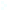Election CitationResearch Interests Name Caflisch, Russel E. Location New York University Primary Field Applied Mathematical Sciences Secondary Field Mathematics Caflisch has made pivotal contributions to applied and computational mathematics for physical systems, including studies of fluid and plasma dynamics. Russ Caflisch's research is on analysis and numerical methods for physical systems, in particular for special solutions and robust numerical methods in singular limits. For kinetics of fluids and plasmas, his results include analysis of the fluid limit and of shock wave solutions for the nonlinear Boltzmann equation, and accelerated simulation methods that are a hybrid of a continuum fluid solver and a Monte Carlo particle solver. For fluid dynamics, he analyzed the development of singularities for vortex sheets, extended this method to the Muskat problem and to a complexified generalization of the incompressible Euler equations, and analyzed the Prandtl equations for a viscous boundary layer. He developed a Brownian bridge method and other adapted and accelerated numerical techniques for quasi-Monte Carlo methods, which are now widely used in finance. He was a leader of a group of mathematicians and materials scientists that developed a level set method and an island dynamics model for epitaxial growth. As a generalization of compressed sensing, he applied techniques of sparsity and soft-thresholding to PDEs and physics.